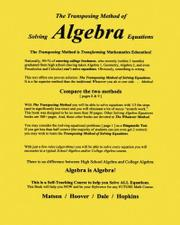The Transposing Method of Solving ALGEBRA Equations

The Transposing Method is Transforming Mathematics Education

by Wayne R MatsonHooverDaleHopkins

Pub Date: Oct. 28th, 2010
ISBN: 978-1453865712
Publisher: CreateSpace

A math professor guides students through a nontraditional way of solving algebraic equations.

In this basic math textbook, Matson (Matsonian Inverse Transposing Method, 2000) introduces readers to his method of solving equations in algebra by transposing the positions of the factors. Several examples demonstrate how the transposing method can produce a result in fewer steps than the more commonly taught method of applying the same operation(s) to both sides of an equation. The book guides readers through both simple and complicated equations as Matson demonstrates that his method works for operations involving exponents as well as those that contain only addition, subtraction, multiplication or division. After covering the fundamentals of the transposing method, the book moves into specific applications of the math involved, from finance to physics. Readers are given the opportunity to take evaluations at both the beginning and end of the book, with all answers provided. Readers are urged to learn to solve equations via the transposing method using graphing calculators, a technology the author strongly endorses. The book concludes with Matson’s theories regarding the shortcomings of K–12 math education in the United States. While Matson’s method of solving equations is an effective one, the book suffers somewhat from formatting choices that can make the lessons difficult to follow. Bold and italic typefaces are sometimes used to excess, and many of the equations are rendered as in-line text, conserving space at the expense of clarity. The concluding section, in which Matson makes his suggestions for improving math education, could benefit from streamlining the prose—“Teachers should teach Math not Tricks. Teaching tricks takes valuable time away from teaching Math. And, none of the students remembers how to use the tricks in college.” The prose section also adds little of value to the lessons he provides far more clearly in the previous chapters, which are likely to be of use to students looking for a simpler way of solving equations.

A logical, uncommon method of solving algebraic equations that may prove useful to students at any level.